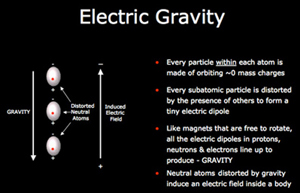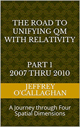# The spatial orientation of gravity and electromagnetism

What makes gravitational force different from those of electromagnetism is that gravity acts along the perpendicular axis of space-time while electromagnetic forces acts in the two-dimensional plane that is perpendicular to gravity.  This is the reason why gravity only acts in one direction attractive while that of electromagnetic can act in two directions, attractive and repulsive because it has the freedom to move along that two dimensional plane.Einstein had difficulty in understanding how derive to the forces of electromagnetic as they moved through space in terms of his space-time model as was documented by the American Institute of Physics.

From before 1920 until his death in 1955, Einstein struggled to find laws of physics far more general than any known before. In his theory of relativity, the force of gravity had become an expression of the geometry of space and time. The other forces in nature, above all the force of electromagnetism, had not been described in such terms. But it seemed likely to Einstein that electromagnetism and gravity could both be explaneed as aspects of some broader mathematical structure. The quest for such an explanation â€” for a unified field theory that would unite electromagnetism and gravity, space and time, all together â€” occupied more of Einstein’s years than any other activity.

However, the reason is NOT that his theories could not support electromagnetism but more likely because time moves only one direction forward similar to how gravity only moves in one direction attractive.  However, electromagnetism “moves” in two direction attractive and repulsive therefore it is difficult to understand how one directional properties of time could be responsible for it.

Yet Einstein gave us an easier way to see how and why his space time model can be linked to the positive and negative forces associated with electromagnetism when he used the constant velocity of light to define geometric properties of forces in a space-time environment  This is because that would allow one to convert a unit of time in his four-dimensional space-time universe to a unit of space in a universe consisting of only four *spatial* dimensions.  Additionally, because the velocity of light is constant it is possible to define a one to one correspondence between his space-time universe and one made up of four *spatial* dimensions.

In other words, by mathematically defining the geometric properties of time in his space-time universe in terms of the constant velocity of light he provided a qualitative and quantitative means of define the time-based components in terms of its equivalent in only four spatial dimensions.

The fact that one can use Einstein’s equations to qualitatively and quantitatively redefine the displacement associated energy in a space-time environment in terms of four *spatial* dimensions is one bases for assuming, as was done in the article  Defining energy? Nov 27, 2007 that all forms of energy including gravitational and electromagnetism can be derived in terms of a spatial displacement in any “surface” or plane of three-dimensional space with respect to a fourth *spatial* dimension.

This allows one to form a physical image of how electromagnetic forces can be both attractive and repulsive in terms of the differential force caused by the “peaks” and “toughs” of an energy wave moving in the three-dimensional plane with respect to a fourth *spatial* dimension that is perpendicular to gravity’s.

For example, Classical wave mechanics tells us a wave on the two-dimensional surface of water causes a point on that surface to become displaced or rise above or below the equilibrium point that existed before the wave was present.  A force is developed by that differential displacement of the surfaces, which will result in the elevated and depressed portions of the water moving towards or become “attracted” to each other and the surface of the water.

Similarly, it tells us an energy wave on the three-dimensional plane with respect to a fourth *spatial* dimension that is perpendicular to gravity would cause a point on that plane to become displaced or “elevated and depressed” with respect to the equilibrium point that existed before that wave was present.

However, it also tells us a force will be developed by those differential displacements in the plane that was perpendicular to gravity that will result in its “elevated and depressed” portions moving towards or become “attracted” to each other as the wave moves through space.

This defines the causality of the attractive forces of unlike charges associated with the electromagnetic field in terms of the force developed by the differential displacements of a point on the three-dimensional plane that is perpendicular to gravity.

However, it also provides a classical mechanism for understanding repelling forces of electromagnetism because observations of water show that there is a direct relationship between the magnitude of a displacement in its surface to the magnitude of the force resisting that displacement.

Similarly, the magnitude of a displacement on a three-dimensional plane with respect to a fourth *spatial* dimension caused by two similar charges will be greater than that caused by a single one.  Therefore, similar charges will repel each other because the magnitude of the force resisting that displacement will be greater for two charges than it would be for a single charge.

One can also derive the magnetic component of an electromagnetic wave in terms of the horizontal force developed by the horizontal displacement caused by its peaks and troughs.  This would be analogous to how the perpendicular displacement of a mountain generates a horizontal force on the surface of the earth, which pulls matter horizontally towards the apex of that displacement.

Additionally, one can derive the causality of electrical component of electromagnetic energy in terms of the energy associated with its “peaks” and “troughs” that is directed perpendicular to its velocity vector while its magnetic component would be associated with the horizontal force developed by that perpendicular displacement because classical Mechanics tells us a horizontal force will be developed by that displacement which will always be 90 degrees out of phase with it.  This force is called magnetism.

This shows that one can use Einstein’s General theory of Relativity to derive the physical properties of both electromagnetism and gravity.  Additionally it defines the reason why the force of gravity only acts only by attracting objects is because it is confined to the perpendicular axis of space-time or its equivalent in four *spatial* dimensions while electromagnetism can both, attract and repulse objects because it has the freedom to move objects or particles two directions in the two dimensional plane that is perpendicular to gravity’s .

It should be remembered that Einstein’s genius allows us to choose whether to define an electromagnetic wave either a space-time environment or one consisting of four *spatial* dimension when he defined its geometry in terms of the constant velocity of light.

Later Jeff

 The Road to Unifying QM with Relativity part 1 2007 thru 2010Ebook \$8.00Paper Back \$15.00 The Road to Unifying QM with Relativity part 2 2011 thru 2014Ebook \$8.00Paper Back \$16.00 The Road to Unifying QM with Relativity part 3 2015 thru 2020Ebook \$8.00Paper Back \$18.00